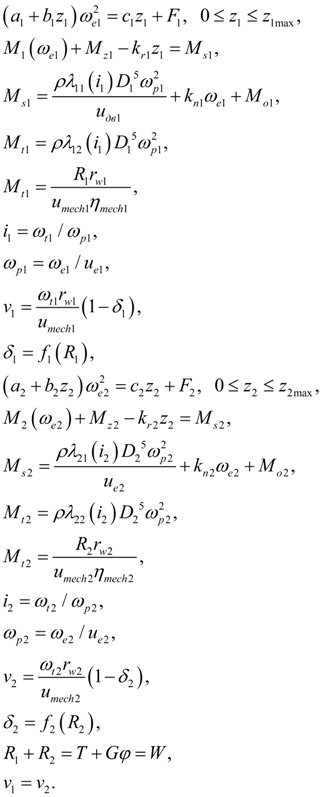Contents >> Engineering Mathematics >> Mobile Machines >> Traction Analysis >> Two engines transmission >> System of equations

 Traction analysis of hydro mechanical transmissions - System of equations of double-motor transmission System of the equations Calculation of traction characteristics of double-motor hydro mechanical transmission of the wheel machine is reduced to the solution of the following system of nonlinear equations (1) considering the external characteristics of diesel engines with centrifugal regulators, the characteristics of hydraulic torque converter and the slipping characteristics of wheel carrier. Here besides the equations similar (1), the equations of traction balance of bridges forces and the condition of equality of speeds of forward movement for each bridge are added :(10) Here the same designations of coefficients and unknowns, as for the single-motor scheme are accepted, and indexes 1 and 2 concern to different branches of two-motor transmission.

 < Previous Contents Next >